The PHREG Procedure

Schemper-Henderson Predictive Measure

Measures of predictive accuracy of regression models quantify the extent to which covariates determine an individual outcome. Schemper and Henderson’s (2000) proposed predictive accuracy measure is defined as the difference between individual processes and the fitted survivor function.

For the ith individual (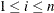), let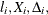and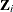be the left-truncation time, observed time, event indicator (1 for death and 0 for censored), and covariate vector, respectively. If there is no delay entry, then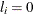. Let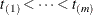be m distinct event times with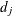deaths at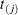. The survival process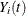for the ith individual isLet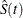be the Kaplan-Meier estimate of the survivor function (assuming no covariates). Let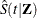be the fitted survivor function with covariates, and if you specify TIES=EFRON, thenis computed by the Efron method; otherwise, the Breslow estimate is used.

The predictive accuracy is defined as the difference between individual survival processesand the fitted survivor functions with (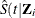)) or without () covariates between 0 and, the largest observed time. For each death time, define a mean absolute distance between theand theas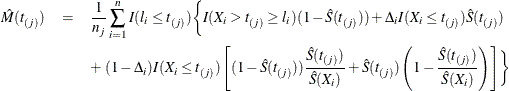where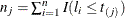. Let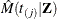be defined similarly to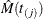, but with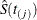replaced by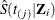and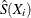replaced by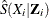. Let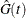be the Kaplan-Meier estimate of the censoring or potential follow-up distribution, and let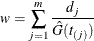The overall estimator of the predictive accuracy with (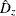) and without (
) covariates are weighted averages ofand, respectively, given by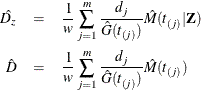The explained variation by the Cox regression is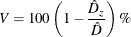Because the predictive accuracy measuresandare based on differences between individual survival processes and fitted survivor functions, a smaller value indicates a better prediction. For this reason,andare also referred to as predictive inaccuracy measures.